# Square root by hand vs Go `math.Sqrt`

Several weeks ago I noticed a video by Matt Parker from @standupmaths talking about “how to find the square root of x by hand”. This was a supplemental video of “calculating π by hand” done as part of π day.

This video brought back college memories of my first semester at the university, I failed Algebra I and the professor Juan A. López mentioned that the second chance exam could include a problem that required finding the square root of x, claiming that it required knowledge from the whole semester. He then gave us a sheet of paper with an algorithm to follow.

I took the second chance exam, I passed, no square root algorithm was required. At the time, I was pissed because the algorithm is quite complex when you are just following instructions without really knowing why you do what you do. To me it was wasted time that I could have used to study other subjects in the exam.

Fast forward 11 years, and I finally understand what the hell was going on. The algorithm that seemed very complex finally made sense, it’s actually very simple and consists on eyeballing a number such that the square of that number is less than x. Then, the difference is how far you are from the real answer, and you repeat on the small square that pops out as the “error”.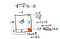Using the algorithm described by Matt Parker, we get an extra little square inside our big square which represents the “error” which we try to minimize.

However… I didn’t understand what Matt Parker was explaining and I came up with my own solution using the same approach, I spread the remaining value on all three remaining areas instead of only the two rectangles and calling the little square an error.

This resulted in a “nice” quadratic equation.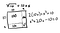Given that I didn’t understand Matt’s algorithm I came up with my own version with a quadratic equation.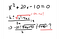I just realized that calculating the square root is a recursive problem.

Solving this quadratic equation ends up in having to find the square root of a larger number. The process repeats itself forever because imperfect roots are irrational numbers, so we keep going until our desired precision.

Now… the point of all if this is finding the root of x by hand. I tried finding the root of a couple numbers up to a 3 decimal point precision, and it was very easy but laborious.

Time to experiment!

`func sqrt(n int64) float64 {  closest := closestInt(n) // Binary search, ints only, no cheating!  x:= quadraticEq(-2*closest, closest*closest-n)  return float64(closest) - x}// ( -b - sqrt(b²-4ac) ) / (2a)func quadraticEq(b, c int64) float64 {  diff := 4 * c  disc := b*b - diff  if p := float64(diff) / float64(disc); p <= precision/42 {    return p  }  discSqrt := sqrt(disc)  return (float64(-b) - discSqrt) / 2}`

I wrote some tests and benchmarks, and looked at the results.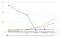Newton-raphson and binary search grow logarithmically, standard implementations run in constant time, and the “by hand” method… grows faster?!

From the results, I observed that:

• The standard Go implementations run in constant time. They are actually bound by the number of bits which is a constant for practicality.
• Binary search and Newton-Raphson as expected grow logarithmically. I thought Newton-Raphson would be faster because it does some kind of interpolation search.
• The “by hand” algorithm becomes faster with larger numbers, wait what?!

I reviewed my algorithm, it is a recursive algorithm that runs binary search on every step. It runs until my desired precision is reached, or is it?

Turns out that I can’t actually check the precision of the square root, instead I’m comparing the ratio of `b*b` vs the discriminant (`b*b-4*a*c`); however, while taking a closer look I realized I could simplify the equation much more!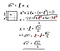From the quadratic equation, I simplified until I got sqrt(n) = sqrt(4n) / 2
`func sqrt(n float64, pow2 uint) float64 {  // Approximation to end recursion  if n > 1<<50 {    closest := closestInt(n)    return closest / float64(int64(1)<<pow2)  }  // f(n) = f(4n) / 2  return sqrt(4*n, pow2+1)}`

Now, I know what you are thinking, the `4` can be pulled out as a `2` which “cancels out” with the divisor. We arrive at `sqrt(n) = sqrt(n)`, what a disappointment. Eppur si muove, so why does it work?

Expressing all this algebra in function notation, `f(x) = f(4x) / 2`, and by using a mathematical induction-like strategy (which you can prove yourself), I arrived at this function: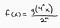f(x): square root of xg(x): largest integer such that g(x)² <= xn: arbitrary number, larger n gives more precise results

Better yet, this can be generalized even further so it works for any n-th root and you don’t need to use `4` and `2`, any constant and its square can be used to wash out the error of the approximation. You probably get the idea, but `g(x)` finds an approximation, which is inherently wrong, by definition it contains an error factor. `4` is attached to `x` and now the error is proportional to the square root of `4`, i.e. `2` and that’s why we divide by `2`.

For us, humans who learned a decimal numeric system, we could instead use `100` and `10` to make it simpler, and this works in any numeric base! We’ll keep using `4` and `2`, since that’s `100` and `10` in binary which is what the computer understands best.

Finally we arrive at this general formula on how to get nth-root of x by hand, for any root degree: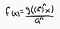m: root degreef(x): m-th root of xg(x): largest integer such that g(x)^m <= xn: arbitrary number, larger n gives more precise resultsa: arbitrary number to wash out approximation error

Woah! How did I get here and what was I even trying to write in the first place?

Oh, right! Square root by hand vs Go’s implementation. We’ve come a long way, but finally we can implement a “by hand” algorithm that runs much faster than what we had before, so how does it fare against the others?

`func sqrt(n float64) float64 {  n = n * float64(factor)  return closestInt(n) / divisor}`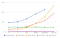The “by hand” method now grows logarithmically but performs worse than other divide & conquer algorithms.

The algorithm runs much faster than the previous by-hand method. Not only for the computer, but doing this by hand should also be a piece of cake. It still grows logarithmically because I’m using binary search to find an approximation.

I tried a couple different approaches like using `big.Int` and `float64` to avoid having to deal with `int64` overflow, but in the end they performed incredibly worse so I didn’t think they were worth adding here.

I tried looking for this algorithm but I couldn’t recognize it from this list on Wikipedia. I may just be bad at searching, but maybe I just discovered a new algorithm! (I doubt it).

Anyways, thanks for staying with me this far! My code has been uploaded to GitHub so you can judge for yourself.

Written by

## Goodwine Carlos

#### Software engineer at Google. Interested in technology, science, videogames, fantasy, and anime. Views are my own.

Welcome to a place where words matter. On Medium, smart voices and original ideas take center stage - with no ads in sight. Watch
Follow all the topics you care about, and we’ll deliver the best stories for you to your homepage and inbox. Explore
Get unlimited access to the best stories on Medium — and support writers while you’re at it. Just \$5/month. Upgrade